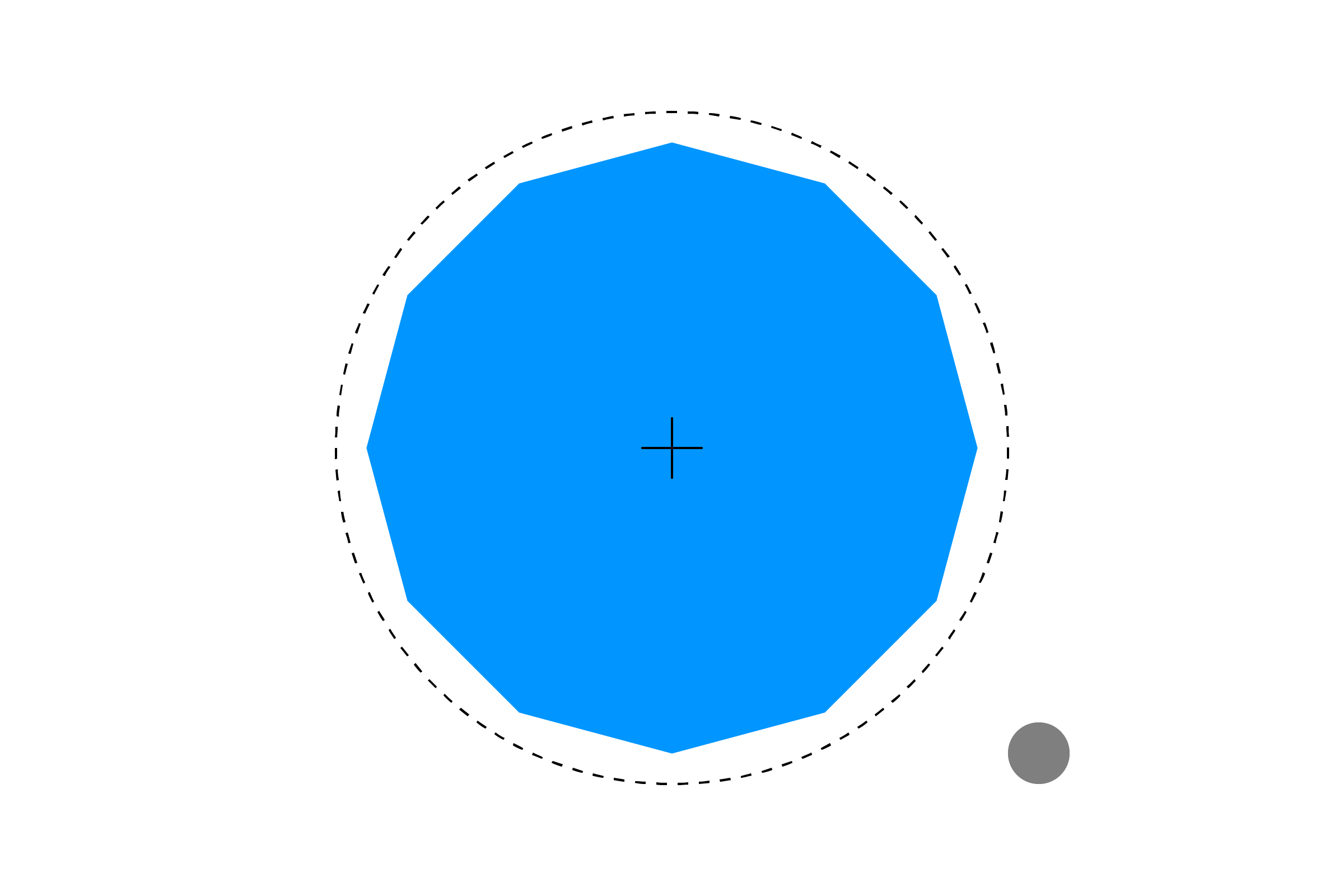[ your browser does not support the canvas tag ]

# CIRCLE/CIRCLE

Collision with points is fine, but rarely do objects actually occupy a single point in space. Next, we can use the same application of the Pythagorean Theorem from the Point/Circle example to test if two circles are colliding.

First, calculate the distance between the two circle's centers:

``````float distX = c1x - c2x;
float distY = c1y - c2y;
float distance = sqrt( (distX*distX) + (distY*distY) );
``````

To check if they are colliding, we see if the distance between them is less than the sum of their radii.

``````if (distance <= c1r+c2r) {
return true;
}
return false;
``````

Built into a full example, it looks like this:

``````float c1x = 0;      // circle 1 position
float c1y = 0;      // (controlled by mouse)
float c1r = 30;     // radius

float c2x = 300;    // circle 2 position
float c2y = 200;
float c2r = 100;

void setup() {
size(600,400);
noStroke();
}

void draw() {
background(255);

// update position to mouse coordinates
c1x = mouseX;
c1y = mouseY;

// check for collision
// if hit, change color
boolean hit = circleCircle(c1x,c1y,c1r, c2x,c2y,c2r);
if (hit) {
fill(255,150,0);
}
else {
fill(0,150,255);
}
ellipse(c2x,c2y, c2r*2,c2r*2);

// other circle, controlled by mouse
fill(0, 150);
ellipse(c1x,c1y, c1r*2,c1r*2);
}

// CIRCLE/CIRCLE
boolean circleCircle(float c1x, float c1y, float c1r, float c2x, float c2y, float c2r) {

// get distance between the circle's centers
// use the Pythagorean Theorem to compute the distance
float distX = c1x - c2x;
float distY = c1y - c2y;
float distance = sqrt( (distX*distX) + (distY*distY) );

// if the distance is less than the sum of the circle's
// radii, the circles are touching!
if (distance <= c1r+c2r) {
return true;
}
return false;
}
``````

Circle/Circle collision can be used to create "bounding circles" around more complex objects. While sacrificing accuracy, this kind of collision detection is very fast and can be a good approximation.You may be wondering why we are only talking about circles and not ellipses. It might seem fairly similar, but the math for ellipse collision is actually quite complicated. Consider it a great challenge once you master the other collision examples!

NEXT: Section 1 Challenges